﻿ One Dimensional Motion | Zona Land Education

# One Dimensional MotionOne dimensional motion is motion along a straight line.

The line used for this motion is often the familiar x-axis, or x number line. The object may move forward or backward along this line: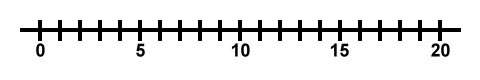Forward is usually considered positive movement, and this movement is usually considered to be to the right. So, as an object moves forward down the x-axis, it is heading toward larger and larger x coordinates, and we say that it has a positive displacement and a positive velocity: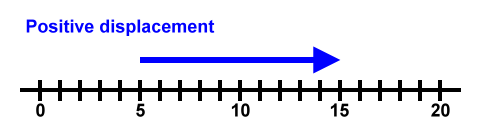Backward is usually considered negative movement to the left. As an object moves backward along the x-axis, it is heading toward smaller and smaller x coordinates, and we say that it has a negative displacement and a negative velocity: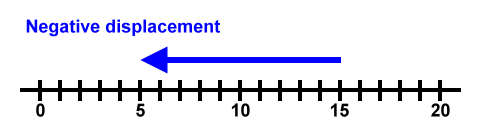Here is an animated diagram showing an object moving along with a constant positive velocity. (See the 'Code' button near the bottom of the page if you want to examine the animation computer code.)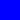### One Dimensional, Constant Velocity Motion

• An object moves with constant velocity.
• Its speed does not change.
• Its direction does not change.
• It moves over equal distances during equal time intervals.

In the above animation we say that the displacement of the object is positive because the object's motion is toward greater and greater x-coordinates. That is, for any segment of the motion the later x coordinate, called x2, is greater than the former x coordinate, called x1. This makes the displacement, calculated with (x2 - x1), a positive value. It works like this:

• The object starts out somewhere, let's call it x1, and x1 is at 2 m. That looks like this: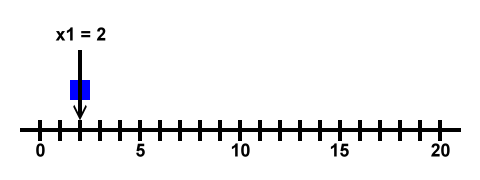• The object moves and gets to x2, and x2 is at 5 m, as: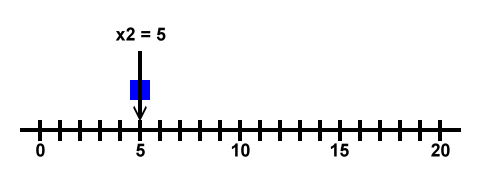• The object is said to have covered a displacement of, or was displaced, or has a displacement of....
• (x2 - x1) = (5 m - 2 m) = 3 m. Here's the picture: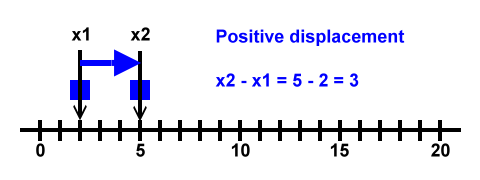In the above animation we say that the velocity is positive, ultimately, because the displacement is positive, and velocity follows the direction of the displacement. Another way to think about it is that conventionally movement to the right is considered to be a positive velocity, but that does not get to the root of it. The velocity is positive because the change in the x-coordinates is positive

Also, in the above animation notice that the object moves through equal distances during equal time intervals. This is what we mean by a constant velocity.

Here is another animated diagram showing an object moving along with a constant positive acceleration: (See the 'Code' button near the bottom of this page if you want to examine the computer code that runs this animation.)### One Dimensional, Constant Acceleration Motion

• An object moves with constant acceleration.
• Its speed increases as time passes.
• Its direction does not change.
• It moves over ever greater distances as equal time intervals pass.

In the above animation we notice that the object moves faster and faster as it goes from left to right. This speeding up is one type of acceleration. Notice that the object covers more distance in the later time intervals than in the early ones. This is because in the later time intervals it is traveling faster.

An acceleration happens when an object's velocity is changed as time passes.

Changes in velocity can happen in a couple of ways.

• If an object speeds up or slows down, its velocity is said to change.
• Also, if the motion of an object changes direction, its velocity changes.

Now, in one dimensional motion the object will not change direction. It's moving in a straight line. So, for one dimensional motion, changes in velocity can only occur by a speeding up or a slowing down. It follows that for one dimensional motion, accelerations can only occur over the time intervals during which the object speeds up or slows down.

In other words, for one dimensional motion, the only way an object can accelerate is to speed up or slow down.

You can examine the computer code used in the two animations on this page by clicking the following button:

Here's the code that runs the above animation.

You can change the code, if you like, and then click the following 'Reevaluate code' button. The program will then work as per your changes. Of course, your changes, especially random changes, can introduce errors, miscalculations, and browser crashes. If you need to get things back to their original condition, just reload this page using your browser's reload button.

The intention here is to conveniently show the inner workings of this program so that you understand how the diagram is drawn. Can you figure out how to make the constant velocity object move faster? Can you figure out how to make the accelerating object accelerate more?

Click the 'Code' button again to close this section.

Custom Search